## Introduction

Prerequisites: Queue

Source on GitHub

Heaps are data structures that can pop the maximum or minimum value or push a value very efficiently. Heaps are special binary trees which have the property that: the value of each node is greater than the values of all its children (a max heap) or the value of each node is less than the values of all its children (a min heap). Priority queue's are most efficiently implemented as heaps. This guarantees that the maximum or minimum element is the root node.

Heaps store their data level by level in a binary tree. This allows us to store heaps in an array. The root index is 0. For every node with index i, the left index can be found by using the formula 2*i+1 and the right index can be found by using the formula 2*i + 2. The parent of a node can be found by integer division as (i-1)/2.

```root = 0
leftChild = index * 2 + 1
rightChild = index * 2 + 2
parent = (index - 1)/2
```

Indexes of a heapExample Heap: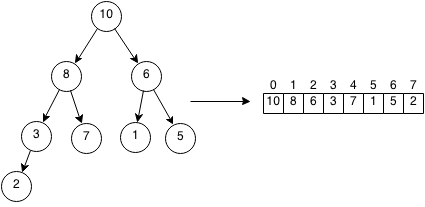A heap has two operations: push and pop. Pushing an element into a heap adds it into the heap while ensuring that the properties of the heap still hold. Popping removes an element from the top of the heap and the heap needs to ensure that the properties of the heap still hold.

Operation Resize Push Pop Heapify
Time Complexity O(n) O(log n) O(log n) O(n)

## Class

We will implement a max heap in Java and in our class we need to store the elements in the heap and the size of the heap.

```public class Heap {

public int[] arr;
public int size;

public Heap(int startSize) {
arr = new int[startSize];
size = 0;
}
}
```

## Resize

When the heap reaches capacity, we need to resize it to be able to contain more elements.

```public void resize() {
int[] newArr = new int[arr.length * 2];
for (int i = 0; i < size; i++) {
newArr[i] = arr[i];
}
arr = newArr;
}
```

## Swap

Swap will switch two nodes in the heap.

```public void swap(int a, int b) {
int tmp = arr[a];
arr[a] = arr[b];
arr[b] = tmp;
}
```

## Push

Pushes the number x into the priority queue. We can do this by adding it to the bottom of the heap and then keep swapping it upwards if it is greater than the parent.

Example:Add 9 to the end of the heap.The parent of 9 is 3 and smaller so we can swap the two.The parent of 9 is 8 and smaller so we can swap the two.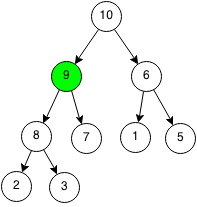Since the parent of 9 is 10 and greater than 9 then we can stop. We can also see that the heap structure is still valid.

```public void push(int x) {

if (size >= arr.length) {
resize();
}

// Insert to the end of the heap.
arr[size] = x;
size++;

int idx = size - 1;
int parent = (idx - 1) / 2;

// Push the node up until the parent is larger.
while (idx > 0 && arr[parent] < arr[idx]) {
swap(parent, idx);
idx = parent;
parent = (idx - 1) / 2;
}
}
```

## Pop

Popping removes the greatest element in the heap. Since the root is guaranteed to be the greatest element as a property of a heap, we remove it and return that element. After removing the root, we replace it with the element at the bottom of the heap and we can keep swapping it with its children until the heap property is satisfied.

Let's do an example of where we pop from a heap. We want to remove the root of the heap and replace it with the last element of the heap.We switch the node and replace it with the last element in the heap.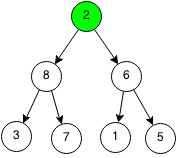The largest child is the left child of 8 and it is larger than the current node, so we swap them.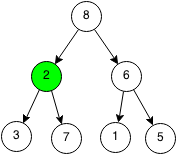The largest child is the right child of 7 and it it larger than the current node, so we swap the nodes again.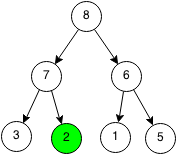When the current node is larger than both children or the current node is at the bottom, then we can stop.

```public void bubbleDown(int idx) {
while (idx < size) {
int left = idx * 2 + 1;
int right = idx * 2 + 2;
// If both child exists.
if (left < size && right < size) {
// If left child is larger than right child and current node.
if (arr[left] > arr[right] && arr[left] > arr[idx]) {
swap(left, idx);
idx = left;
}
// If right child is larger or equal than left child and current node.
else if (arr[right] >= arr[left] && arr[right] > arr[idx]) {
swap(right, idx);
idx = right;
}
// If no children, stop.
else {
break;
}
}
// If there is only a left child.
else if (left < size) {
swap(left, idx);
idx = left;
}
// If there is only a right child.
else if (right < size) {
swap(right, idx);
idx = right;
}
else {
break;
}
}
}

public int pop() {
if (size == 0) {
return 0;
}
// Swap root and last element of heap.
int ret = arr;
arr = arr[size - 1];
size--;

// Push the root down until parent is greater than children
bubbleDown(0);

return ret;
}
```

## Heapify

Heapify takes an array of N elements and transforms it into a heap in the same array. The runtime of heapify is suprisingly O(n) and the proof of that is beyond the scope of this book.

```public void heapify(int arr[]) {
this.arr = arr;
// Reach height of tree.
for (int i = 0; i < Math.floor(arr.length / 2.0); i++) {
// Iterate through array.
bubbleDown(i);
}
}
```

## Exercises

1. Implement a min heap in Java.
2. Write a function that checks if an array is a heap.
3. Prove that heapify is O(n).